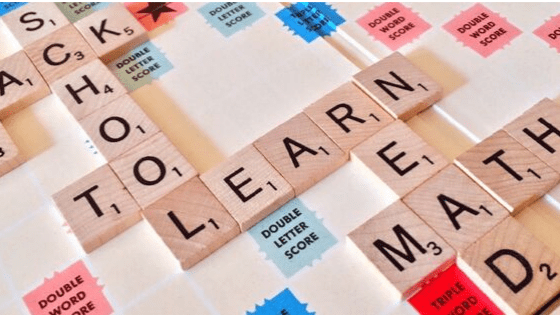# 15 math phrases students should knowWhen it comes to applying mathematics concepts studied in class to the real world situations, many students feel they mostly get confused when translating math concepts and formulas into the corresponding language, specific math words and phrases, that we use every day but do not make immediate conscious connection to the math behind it. We just “know” it means this or that, without thinking what it would look like in terms of math formulas, expressions or equations.

Let’s take a look at 15 math words and phrases that will improve every student’s comprehension of math concepts when applied to real life situations.

## 1. Two more than three

When one quantity is greater than the other by a certain amount, we use the word “more” and it represents addition.

3 + 2 = 5

## 2. Three less than five

When one quantity is smaller than the other by a certain amount, we use the word “less” and it represents subtraction.

5 – 3 = 2

## 3. Two times (twice) eleven

When one quantity is double the other, we use “two times” or “twice”, which represents multiplication by two.

11 x 2 = 22

## 4. Half a dozen

Half means division of a given value by two.

12 / 2 = 6

## 5. Variable

We use variables to represent any number. Variables are letters of the alphabet used in mathematical formulas, expressions and equations. Variables are also used in coding. Online math games would not exist if their creators did not know how to operate the variables.

The word “variable” is derived from the verb “to vary” which means “to change depending on the situation”.

5m + 3n

## 6. Expression

A mathematical (algebraic) expression is a combination of numbers and variables put together with the help of operations into one mathematical sentence. Algebraic expressions are often used to represent certain quantities.

Expressions are different from equations.

We do not “solve” expressions and they do not contain an equal sign.

25x + 3y

## 7. Equation

An equation is an equality of two algebraic expressions containing a variable, which is the unknown. The purpose of solving equation is to determine the unknown value by relating two quantities to each other in a meaningful way. In Canada, more attention is given to solving linear equations in grade 7 math and grade 8 math courses.

3m + 1 = 2 (m – 4)

## 8. Difference/sum of the two numbers

Difference is the result of subtracting one value from another.

Sum is the result of adding the two values.

These words are frequently used in grade 6 EQAO test, as well as online mental math practice (Prodigy Game).

3 – 2 = 1 3 + 2 = 5

## 9. Product/quotient of the two numbers

Product is the result of multiplying the two values.

Quotient is the result of dividing one value by another.

2 x 3 = 6 6 / 3 = 2

## 10. Rate

A rate is a ratio between two related quantities with different units.

Rate is frequently used when applying math to real world situations.

The most common example of rate is Speed (km/h).

Khan Academy has a number of free math videos involving rate.

## 11. To prove

Sometimes we are being asked to demonstrate mathematically (frequently by the method of substitution) that something makes sense. This means “to prove” something. When teaching middle school, it is important that teachers introduce this word regularly in math classes.

## 12. Odd/even

Odd numbers are whole numbers that start with a 1 and continue up or down the number line skipping every other number.

Even numbers are whole numbers that start with a 2 and continue up or down the number line skipping every other number.

1, 3, 5,….. 2, 4, 6, 8,……

## 13. To substitute

When applying math concepts to real life situations we often need to represent numbers as variables or one expression in terms of another expression. The process of replacing a variable with a number or replacing one part of the expression/equation with another is called “substitution”.

### If the cab traveled 20 km, what is the cost of the trip?

Substitute 20 in place of d and evaluate.

## 14. Dimensions

Length, width and height are dimensions.

When we are being asked to find the dimensions, we are being asked to determine the length, width or height depending on the shape.

## 15. Complete solution

When trying to figure out a real-life mathematical problem, it is important to record the solution in a well structured and organized manner, include all required steps and statements.

Such components as “let” and “therefore” statements, equations, formulas and diagrams make the solution complete.

0